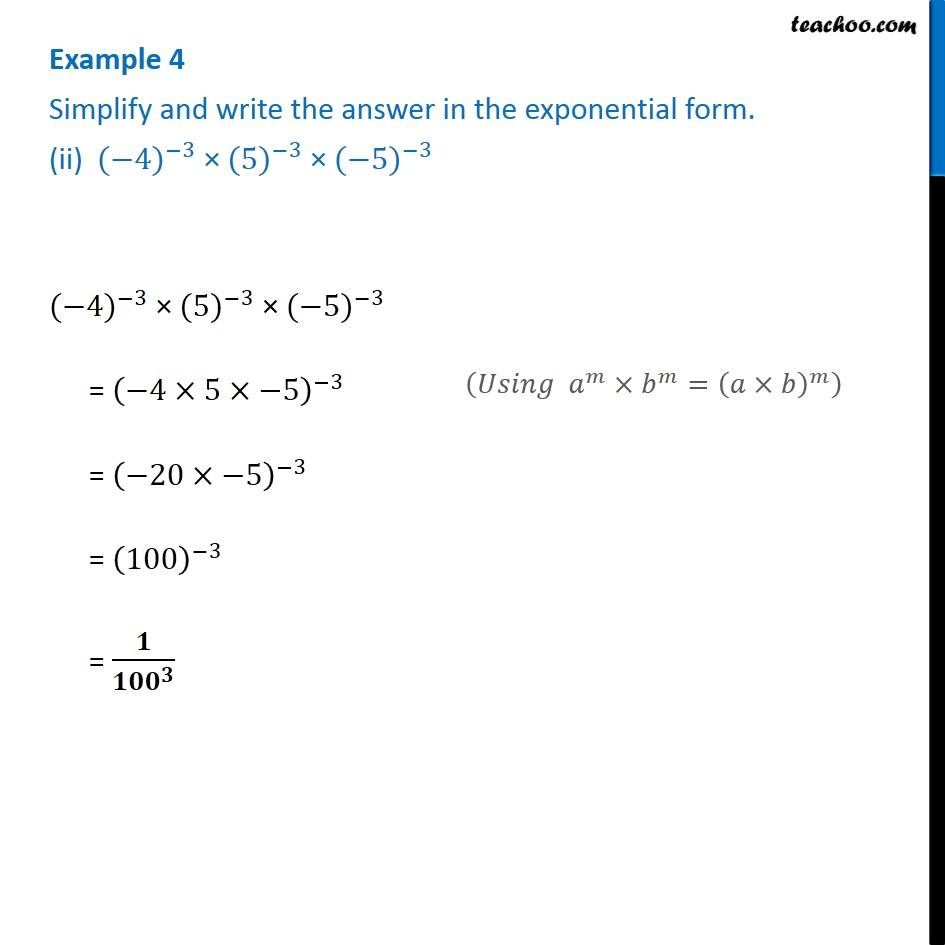Examples

Chapter 12 Class 8 Exponents and Powers
Serial order wiseGet live Maths 1-on-1 Classs - Class 6 to 12

### Transcript

Example 4 Simplify and write the answer in the exponential form. (ii) 〖(−4)〗^(−3) × 〖(5)〗^(−3) × 〖(−5)〗^(−3) 〖(−4)〗^(−3) × 〖(5)〗^(−3) × 〖(−5)〗^(−3) = (−4×5×−5)^(−3) = (−20×−5)^(−3) = (100)^(−3) = 𝟏/〖𝟏𝟎𝟎〗^𝟑 (𝑈𝑠𝑖𝑛𝑔 𝑎^𝑚×𝑏^𝑚=(𝑎×𝑏)^𝑚 )